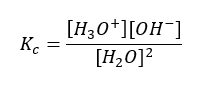# Concept of pH

Equilibrium: Concept of pH

The pH of a solution is used to measure how acidic or basic a solution is. A solution that has a pH value of exactly 7 is neutral—neither acidic nor basic. A solution is basic when pH > 7 and acidic when pH <7. Bases contribute OH ions when they dissociate and bind to the H ions produced when water dissociates. In acidic solutions, the protons that are released into solution will notremain alone due to their large positive charge density and small size. They are attracted to the negatively charged electrons on the oxygen atoms in water, and form hydronium ions. The reaction between an H+ (H3O+) ion from the acid and the OH ion from the base to form water, H2O causes neutralization.

Strong acids ionize completely in aqueous solution. There are more H3O+ ions than OH ions in the solution.

HCl(aq) + H2O H3O+(aq) + Cl(aq);

In solutions of strong monoprotic acids HA, such as HCl and HClO4,

[H3O+] = [HA]0

For example, in 0.10 M HCl, [H3O+] = 0.10 M, and pH = -log(0.10) = 1.00

Weak acids ionize only partially in aqueous solution. Recall that [H3O+] and pH can be calculated from its Ka value.

HF(aq) + H2O ⇌ H3O+(aq) + F(aq);

For example, in 0.100 M acetic acid, CH3COOH, with Ka = 1.8 x 10-5

Strong bases ionize completely in aqueous solution. There are more OH ions than H3O+ ions in the solution.

NaOH(aq) Na+(aq) + OH(aq);

In a base solution such as 0.050 M NaOH, [OH] = [NaOH]0 = 0.050 M; pOH = -log(0.050 M) = 1.30; pH = 14.00 – 1.30 = 12.70

Weak bases ionize only partially in aqueous solution. [OH] and pH can be calculated from the initial concentration of the base and its Kb value.

NH3(aq) + H2O NH4+(aq) + OH(aq);

Pure water is neither acidic nor basic. It is neutral and undergoes autoionization. Water ionizes to produce hydronium ion, H3O+ and hydroxide ion, OH, thus it has both acid and base properties. Kw is called water ionization constant.The neutralization reaction is exothermic and releases approximately 56 kJ per mole of acid and base.Kw == [H3O+][OH] = 1.0 x 10-14 at 25oC

pOH = -log[OH]

pKw = -log(Kw); pKa = -log(Ka); pKb = -log(Kb)

Pure water at 25oC: [H3O+] = [OH] = 1.0 x 10-7M.

-log(Kw) = -log [H3O+] + (-log[OH])

pKw =pH + pOH = 14.00. At 25oC, pOH = 14 – pH

When one unit of pH is decreased, it represents a 10x increase in H+ concentration. Eg: pH 4 is ten times more acidic then pH 5. Eg: pH 10 is ten times more basic then pH 9.

pH=-log [H+] ; where, [H+] = concentration of the acid or hydronium ion

Eg. 0.01M HCl has a pH of?

This means you have 0.01 moles of H+and .01 moles of Cl per every 1 L

Hence, pH = -log(0.01) =2Fig : Relationship between the acidity of a solution and pH.